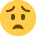Current updates regarding coronavirus (Covid-19) and the precautions AAT are taking will be continually updated on the below page.

We hope you are all safe and well and if you need us we will be here. 💚

# Formula's for financial performance efficiency,capacity and activity ratios

The book says formulae for the above will be given in assessment, however, our tutor has stressed that we all learn it. Has anyone out there who has done this exam can shed a light on this. Is it given or do you have to know this well? Also ROCE and RONA formulas do you have to learn that too! I would appreciate if someone could let me know. finances variances are difficult as it is!!• Dear Zippi
The crucial document for your question is this: https://www.aat.org.uk/study-support/resource/file/49226
It is the guidance notes for the unit and has been written by the examiner.
It spells out precisely what can be tested. It also shows the examiner's interpretation of ratios that might be different to those of other examiners or some text books. Ratios are used to help assess business performance, rather than somethings invented to trouble you in exams. In some businesses a ratio might be used in a different way (using a different formula) than in another. The examiner provides clarity in terms of what this exam expects.

1) Financial (profitability, liquidity, efficiency and gearing)
a. Gross profit margin, = gross profit/sales revenue x 100%
b. Profit margin, = profit/sales revenue x 100%
c. Administration costs as a percentage of revenue, any cost as a percentage of revenue = cost/sales revenue x 100%
d. Current ratio = current assets/current liabilities
This can be expressed as a number only or as a number: 1, for example if current assets are £10,000 and current liabilities are £8,000 the ratio is 1.25 or 1.25:1. In questions students should just use 1.25 as their answer.
e. Quick ratio = (current assets less inventory)/current liabilities and again should be expressed as a single number in assessments.
f. Trade cycles (receivable days = receivables/revenue x 365, inventory days =
inventory/cost of sales x 365, payable days = payables/cost of sales x 365)
g. Gearing ratio can be calculated as either total debt/(total debt plus equity) x 100% or total debt/total equity x 100%. Total debt must include both long term and short term debt.
Both computer marked and human marked tasks will allow both calculations.
h. Value added = revenue less the cost of materials used and bought in services

2) Efficiency, capacity and activity ratios (the formula for these ratios will be provided in assessment questions)
a. Labour efficiency ratio = standard hours for actual production/actual hours worked expressed as a percentage.
b. Capacity ratio = actual hours worked/budgeted hours expressed as a percentage.
c. Labour activity ratio = standard hours for actual production/budgeted hours, or actual output/budgeted output expressed as a percentage.

3) Indicators to measure efficiency and productivity
a. Measures of efficiency include ROCE or RONA, profit margin and efficiency ratio for labour ROCE = Return/capital employed, in tasks return will be equal to the profit in the statement of profit or loss (income statement). This ratio is always expressed as a percentage.
RONA = Return / net assets, return will be equal to the profit in the statement of profit or loss (income statement). This ratio is always expressed as a percentage.
b. Productivity measures are likely to be measured in units of output, or related to output in some way. Examples include number of, say, vehicles manufactured per week, operations undertaken per day, passengers transported per month, units produced per worker per day, rooms cleaned per hour or meals served per sitting.

4) Indicators to measure quality of service and cost of quality
a. The number of defects/units returned/warranty claims/customer complaints, the cost of inspection/ repairs/re-working,
b. Prevention costs, appraisal costs, internal failure costs, external failure costs.

5) Students may be asked to compare given indicators with performance indicators that they have calculated. They may have to undertake a benchmarking exercise and need to understand the purpose of benchmarking.

I hope this clears up your question
1. Learn the main ratio formulas as they will not be provided
2. Expect the Efficiency, Capacity and Activity Ratio formulas to be provided
3. Be ready for industry specific ratios which you have to derive yourself
I recommend Management and Cost Accounting For Dummies
http://eu.wiley.com/WileyCDA/WileyTitle/productCd-1118650492.html
as much of it was written with the AAT Level 4 Financial Performance unit in mind

Sandy

[email protected]
www.sandyhood.com
Sandy
[email protected]
www.sandyhood.com
• Thanks Sandyhood, I appreciate your help but still halfway through chapter 7 therefore don't really know industry specific ratio and how to derive it, I guess when I do some papers it will become clearer. Much appreciated. Sounds a lot I am dreading this one!